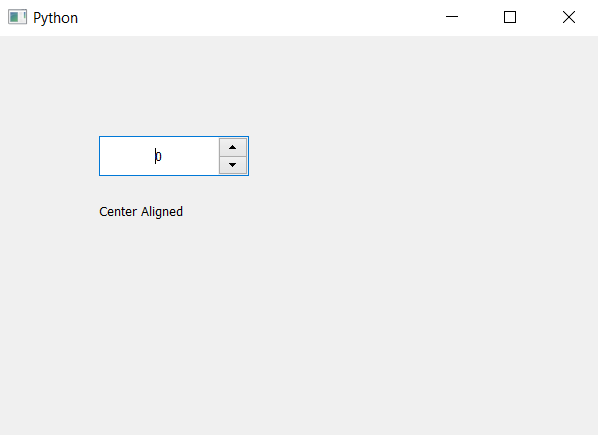Skip to content
Related Articles
PyQt5 QSpinBox – Checking if value is at center
• Last Updated : 06 May, 2020

In this article we will see how we can check if the value appears in the center of spin box or not, when we create spin box the value is at the left hand side in order to make the value in center we have to set its alignment flag to center.

In order to do this we will use `alignment` method.

Syntax : spin_box.alignment()

Argument : It takes no argument

Return : It returns Qt object

Steps for implementation –

1. Create a spin box
2. Set its alignment with the help of `setAlignment` method
3. Create label to show the result
4. Get the alignment with the help of `alignment` method
5. Check if alignment is center then update the label
6. Show alignment is not center show not at center

Below is the implementation

 `# importing libraries``from` `PyQt5.QtWidgets ``import` `*` `from` `PyQt5 ``import` `QtCore, QtGui``from` `PyQt5.QtGui ``import` `*` `from` `PyQt5.QtCore ``import` `*` `import` `sys`` ` `class` `Window(QMainWindow):`` ` `    ``def` `__init__(``self``):``        ``super``().__init__()`` ` `        ``# setting title``        ``self``.setWindowTitle(``"Python "``)`` ` `        ``# setting geometry``        ``self``.setGeometry(``100``, ``100``, ``600``, ``400``)`` ` `        ``# calling method``        ``self``.UiComponents()`` ` `        ``# showing all the widgets``        ``self``.show()`` ` `    ``# method for widgets``    ``def` `UiComponents(``self``):`` ` `        ``# creating spin box``        ``self``.spin ``=` `QSpinBox(``self``)`` ` `        ``# setting geometry to spin box``        ``self``.spin.setGeometry(``100``, ``100``, ``150``, ``40``)`` ` `        ``# alignment``        ``alignment ``=` `Qt.AlignCenter`` ` `        ``# setting alignment to centre``        ``self``.spin.setAlignment(alignment)`` ` `        ``# creating label``        ``label ``=` `QLabel(``self``)`` ` `        ``# setting geometry to the label``        ``label.setGeometry(``100``, ``160``, ``200``, ``30``)`` ` `        ``# getting alignment``        ``get_alignment ``=` `self``.spin.alignment()`` ` `        ``# checking if alignment is centre``        ``if` `get_alignment ``=``=` `Qt.AlignCenter:``            ``text ``=` `"Center Aligned"`` ` `        ``else``:``            ``text ``=` `"Not center aligned"`` ` `        ``# setting text to the label``        ``label.setText(text)`` ` ` ` `# create pyqt5 app``App ``=` `QApplication(sys.argv)`` ` `# create the instance of our Window``window ``=` `Window()`` ` `# start the app``sys.exit(App.``exec``())`

Output :Attention geek! Strengthen your foundations with the Python Programming Foundation Course and learn the basics.

To begin with, your interview preparations Enhance your Data Structures concepts with the Python DS Course. And to begin with your Machine Learning Journey, join the Machine Learning – Basic Level Course

My Personal Notes arrow_drop_up